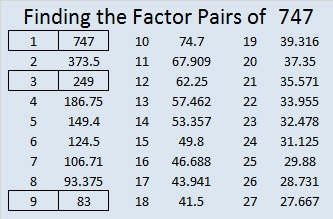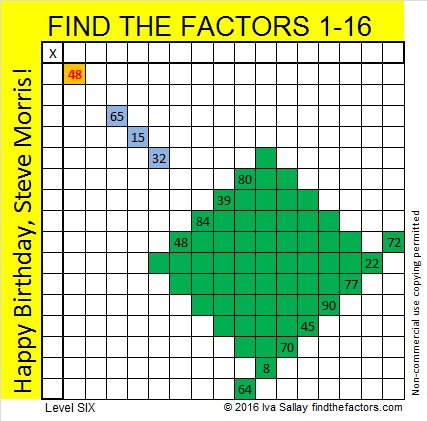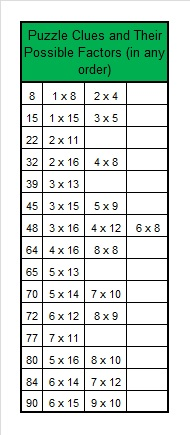# 747 Happy Birthday, Steve Morris!

• 747 is a composite number.
• Prime factorization: 747 = 3 x 3 x 83, which can be written 747 = (3^2) x 83
• The exponents in the prime factorization are 2 and 1. Adding one to each and multiplying we get (2 + 1)(1 + 1) = 3 x 2  = 6. Therefore 747 has exactly 6 factors.
• Factors of 747: 1, 3, 9, 83, 249, 747
• Factor pairs: 747 = 1 x 747, 3 x 249, or 9 x 83
• Taking the factor pair with the largest square number factor, we get √747 = (√9)(√83) = 3√83 ≈ 27.3313007.It’s Steve Morris’s birthday so I thought I’d make him a cake, but no regular size cake will do. He has been one of my earliest supporters, and I know that sometimes even a level 6 puzzle is just too easy for him. Once he sent out this tweet:

Admittedly that puzzle was easier than most level 6’s, but recently he tweeted me a puzzle that I have had to start over more than once and still haven’t conquered:

I think Steve Morris is due for an extra difficult Find the Factors puzzle for his birthday, one that all the numbers from 1 to 16 can be the factors. I’ll wait at least a week before I give any hints to complete it, too. As always, there is only one solution, but it can be found using logic.Print the puzzles or type the solution on this excel file: 10 Factors 2016-01-18

The possible factors for each clue is given below. Adding 14, 15, and 16 as possible factors really complicates the puzzle!—————————————

Now I’ll write a little about the number 747:

747 is a palindrome in base 10. Boeing’s most recognizable airplane also bares that number.

747 can be written as the sum of consecutive numbers five different ways:

• 373 + 374 = 747; that’s 2 consecutive numbers
• 248 + 249 + 250 = 747; that’s 3 consecutive numbers
• 122 + 123 + 124 + 125 + 126 + 127 = 747; that’s 6 consecutive numbers
• 79 + 80 + 81 + 82 + 83 + 84 + 85 + 86 + 87 = 747; that’s 9 consecutive numbers
• 33 + 34 + 35 + 36 + 37 + 38 + 39 + 40 + 41 + 42 + 43 + 44 + 45 + 46 + 47 + 48 + 49 + 50 = 747; that’s 18 consecutive numbers

747 is the sum of three squares six different ways. Three of those ways repeat squares.

• 27² + 3² + 3² = 747
• 25² + 11² + 1² = 747
• 23² + 13² + 7² = 747
• 21² + 15² + 9² = 747
• 19² + 19² + 5² = 747
• 17² + 17² + 13² = 747

—————————————

Since today’s puzzle is the biggest puzzle I have ever published, it could be a little difficult just noticing that a factor had been duplicated in the top row or first column. Here is the tweet Steve Morris sent out once he finally solved the puzzle: